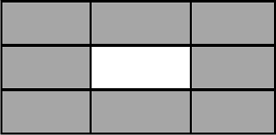# Analytical Reasoning: Trial and Error Method

Questions in the analytical reasoning section test your ability to analyze the situation, reason the cause/ effect and then find a solution. While questions in most of the competitive exams have options with them, but sometimes, we are not presented with options. A lot of effort is required to identify and reach the right option. What works best in such Analytical Reasoning questions is what we call as a trial and error strategy.
What is Trial and Error Strategy?
Try something (Guess), if it does not work, guess again. Over time, our guesses become more educated - and we tend to require fewer trials to reach our solutions.

Tips to remember:
• First of all you need to read the directions of a particular Data Sufficiency question very carefully as examiner can change the directions and even after solving all the questions correctly you mark the wrong answers.
• You need to remember the steps involved in solving a particular Data Sufficiency question and follow them in this particular order: Check A (i.e. the first statement), then Check B (i.e. the second statement) and lastly, if required, combine the two statements to get the answer.
• Do not make any assumptions while solving Data Sufficiency questions.
Solved Questions:
Question: 44 people live in eight houses (3 on each side of the Albert Square). Each house has a different number of people living in it. Each line of three houses has 15 people living in it. How many people live in each house?Solution: Simplifying, we have to get 4 sets of numbers, each of which add up to 15. So start guessing.
The average number of people in a house is 44/8 is roughly 4.5. So what numbers should we take? 2, 3, 4, 5, 6, 7, 8, 9. Now, we should also have an average close to 5 for each set.Let's start:
• Set 1: 3, 5, 7
• Set 3: 2, 4, 9
• Now, the other two sets will have an overlap. So let's guess the overlaps:
• Set 2: Overlaps are 2 and 7. So the third number will be 6. That's great! Because we have not used 6 yet.
• Set 4: Overlaps are 3 and 9. Then the third number ends up to 3 again. Wrong guess! So we need to try again. Overlaps are 3 and 4. In this case third number works out to be 8, which we have not used. Wonderful!
• So here is the answerLearning
• Note that this is not the only solution. There are others possibilities as well.

Analytical Reasoning Practice Question:
Mrs. Choy spent exactly Rs. 10 on 100 eggs for her shop. Large eggs cost her 50 p each. Medium eggs cost her 10 p each. Small eggs cost her 5 p each. For two of the sizes, she bought the same number of eggs. How many of each size did she buy?
Analytical Reasoning - Trial and Error Strategy: Key Learning
Even before guessing the answer, it is important to carefully understand the statement and then narrow down to a set of numbers/ options. These options/ numbers will form a base for using the trial and error strategy.